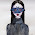Tuesday, January 26, 2010

Delete duplicate rows from Oracle Table:

There are several techniques to delete duplicate rows from a table, but the most effective way is to join the table against itself. Always make sure to select the duplicate data before you delete using below queries.

1. Delete duplicate rows based on one column value using ROWID

SQL> delete from dup_table t1 where rowid > ( select min(rowid) from dup_table t2 where t1.ename = t2.ename);

-- or --
SQL> delete from dup_table t1 where rowid < ( select max(rowid) from dup_table t2 where t1.ename = t2.ename);

2. Use the below query to delete the rows suing Oracle analytic functions

SQL> Delete from dup_table

where rowid in ( select rowid from

( select rowid , row_number() over (partition by col1 order by upper col1 ) row_num from dup_table ) where rno > 1 );

3. You must specify all columns that make the row duplicate in the query, use the below query to delete duplicate records based on two columns or composite unique key

SQL> delete from dup_table t1

where rowid > (select min(rowid) from dup_table t2

where upper(t2.col1) = upper(t1.col1)

and upper(t2.col2) = upper(t1.col2)

);

-- or --

SQL> delete from dup_table t1

where rowid < (select max(rowid) FROM dup_table t2

where t1.col1=t2.col1 AND t1.col2=t2.col2 );

-- or --

SQL> delete from dup_table t1

where rowid <> ( select max(rowid) from dup_table t2

where t2.col1 = t1.col1

and t2.col2 = t1.col2 )

4. If the fields match on the NULL value then duplicate fails to remove the duplicate rows. In this situation add a null check

SQL> delete from dup_table t1
where t1.rowid > ANY (select t2.rowid FROM dup_table t2
where (t1.col1 = t2.col1 OR (t1.col1 is null AND t2.col1 is null))
and
(t1.col2 = t2.col2 OR (t1.col2 is null AND t2.col2 is null))
);

If the table contains duplicate data in upper case and lower case, use below query to delete to delete the data

SQL> delete from dup_table

where rowid in ( select rid from ( select rowid rid, row_number() over (partition by upper(col1) order by upper(col2)) rno from dup_table )

where rno > 1

);

Regards,
Satishbabu Gunukula
http://www.oracleracexpert.com

1.2.